×
INTELLIGENT WORK FORUMS
FOR ENGINEERING PROFESSIONALS

Are you an
Engineering professional?
Join Eng-Tips Forums!
• Talk With Other Members
• Be Notified Of Responses
• Keyword Search
Favorite Forums
• Automated Signatures
• Best Of All, It's Free!

*Eng-Tips's functionality depends on members receiving e-mail. By joining you are opting in to receive e-mail.

#### Posting Guidelines

Promoting, selling, recruiting, coursework and thesis posting is forbidden.

# Deflection Equations2

## Deflection Equations

(OP)
Hey all,

Anyone know of a resource to find a ton of different deflection equations? Everything I can find for a UDL not across the whole beam (and not located starting at a support) only gives the moment equation and no deflection.

Or if you know of a good resource where they go over the derivation of the deflection equations. For weird cases I typically use clearcalcs or RISA 2D for quick results, but it would be nice to have a spreadsheet that uses the superimpose method.

### RE: Deflection Equations

The good old slope deflection equations will get you there. But it can be rather cumbersome to make into a spreadsheet.
The issue with just writing an equation for the deflection of say a simple beam with partial uniform load, is that the the solution is piecewise and constrained. Meaning that the point of maximum deflection, depends heavily on the loading parameters.

The best way to locate the maximum deflection is to use calculus on the deflected shape equation which is derived from slope-deflection differentials equations.
With mathcad or something similar it can be pretty straight forward to set up the triple integral. And solve for maximum displacement.

With excel or something numerical it is quite difficult.

### RE: Deflection Equations

Check out Table 8.1 in Roark's Formulas for some pre-calculated formulas.

In spreadsheet form, using Newmark's "Numerical Procedure for Computing Deflections Moments and Buckling Loads" as a general guide for the method. It was a bit tricky to implement, but works for simple span.

### RE: Deflection Equations

Another option would be to use the direct stiffness method and fixed end moments and such. This involves assuming a shape function for the beam usually cubic. You can get a closed form equation for deflected shape. Not too bad to put this into a spreadsheet.

### RE: Deflection Equations

Ditto to Roark's and use of superposition formulas and symmetry.

### RE: Deflection Equations

#### Quote (driftLimiter)

With excel or something numerical it is quite difficult.
not entirely accurate, excel without some VBA it can be bit of a pain but if you derive everything on a piecewise basis and combine into numerically continuous regions you can solve for the Shear and Slope roots to get the max/min moments and deflections.

Generalized python derivations for Point, Moment, UDL, and linearly varying (Trapezoidal) Loading for Euler-Bernoulli pinned-roller beams can be found here: Link
These were derived via direct integration with the assumption that positive loads act in the negative Y and the beams span in the positive X direction.

### RE: Deflection Equations

(OP)

#### Quote (driftLimiter)

The best way to locate the maximum deflection is to use calculus on the deflected shape equation which is derived from slope-deflection differentials equations.
With mathcad or something similar it can be pretty straight forward to set up the triple integral. And solve for maximum displacement.

I was hoping to be able to solve the integral algebraically and use that formula in the spreadsheet.

### RE: Deflection Equations

(OP)

#### Quote (Celt83)

Generalized python derivations for Point, Moment, UDL, and linearly varying (Trapezoidal) Loading for Euler-Bernoulli pinned-roller beams can be found here: Link
These were derived via direct integration with the assumption that positive loads act in the negative Y and the beams span in the positive X direction.

Is this your toolbox? I see some gifs in there showing functionality, and I've opened it as a .py file but I don't get a GUI as shown in the gifs. Maybe I'm missing something.

### RE: Deflection Equations

yeah, I would (I have) solve the beam in excel. For simply supported beams, with distributed load over any part of the span. Not too hard to extend for beams with 3 supports. Solve the moment and the displacement. With a little thought you may be able to show that max deflection is within the DL limits, and now you don't need to solve the complete beam ... you know the reactions, then you know the beam conditions at the limits of the DL, and with some smarts you can figure it out "easily".

Of course if accuracy is not prized then replace the DL with a point load and have done with it !

"Hoffen wir mal, dass alles gut geht !"
General Paulus, Nov 1942, outside Stalingrad after the launch of Operation Uranus.

### RE: Deflection Equations

You may be looking at my older python library, have not updated it to the newer python version which generally breaks all the GUI stuff.

I originally did the derivations in VBA which can be found here: Link

### RE: Deflection Equations

(OP)
Ah I see, I haven't done much of anything with VBA.

### RE: Deflection Equations

another user here, IDS, has more powerful excel tools: Link

### RE: Deflection Equations

(OP)

#### Quote (dauwerda)

Table 3-23 of the AISC Steel Construction Manual has a decent amount of options.

Yea these are the tables included in the HSC from CISC, but the one section I need (#4. in that table) doesn't provide a deflection equation haha.

### RE: Deflection Equations

There is also the Beam Analysis tool by Alex Tomanovich here:Link

### RE: Deflection Equations

#### Quote (EngDM)

I was hoping to be able to solve the integral algebraically and use that formula in the spreadsheet.

I think you could do this, but it would be a system of equations because the initial conditions that you need for the triple integration (some of them) are dependent on the loading.

### RE: Deflection Equations

Thanks for posting the link Celt83.

https://newtonexcelbach.com/2021/09/28/conbeamu-up...

Regarding finding the maximum deflection, I really think people are overcomplicating things.

Just create reasonably closely spaced output points, then the maximum deflection will be close enough to the actual maximum for all practical purposes.

Or if you really need to be more precise, find the two points with the greatest deflection and subdivide the output over that region.

Doug Jenkins
Interactive Design Services
http://newtonexcelbach.wordpress.com/

### RE: Deflection Equations

#### Quote (IDS)

Regarding finding the maximum deflection, I really think people are overcomplicating things.

Perhaps this is true. Using pre-defined stations (output points) should give a workable result in most cases. A fair point.

### RE: Deflection Equations

(OP)

#### Quote (IDS)

Just create reasonably closely spaced output points, then the maximum deflection will be close enough to the actual maximum for all practical purposes.

Would you not need an equation W.R.T. x for this though?

### RE: Deflection Equations

#### Quote (EngDM)

Would you not need an equation W.R.T. x for this though?

I was assuming using a spreadsheet that allowed the number or position of output points to be specified, but for a simply supported span it's not that hard to set up the calculation from scratch:

1. Calculate the shear diagram.
2. Integrate for bending moment.
3. Assuming zero slope at end 1, integrate twice for slopes and deflections.
4. Find the slope at end 1 so that the deflection at end 2 is zero.
5. Adjust the slopes and deflections along the beam.

Doug Jenkins
Interactive Design Services
http://newtonexcelbach.wordpress.com/

### RE: Deflection Equations

In most cases, finding deflection is an exercise to meet code requirements after having already selected a beam which has adequate strength. Often, the deflections are not a problem. When that is the case, there is no point in wasting design time performing useless work.

In the majority of cases, an engineer should be able to come up with a pretty good approximation with a minimum of effort.

An equal and opposite moment applied to each end of a beam results in a constant moment over the span. Slope and deflection of the real beam are equal to the imaginary shear Vc and bending moment Mc of the conjugate beam. The M/EI curve is the load imagined to be carried by the conjugate beam.

Bending moment for the conjugate beam is Wc*L/8, which is the deflection of the real beam loaded with constant moment across the span.
Wc*L/8 = M/EI*L2/8 = M/EI*L2/8 = WL/8EI*L^2/8 = wL4/64EI.

With a little practice, an engineer should be able to estimate deflections quite accurately. In those rare cases where it is necessary, one must sharpen the pencil a bit.

### RE: Deflection Equations

Blodgett books have several beams with deflections

Design of Welded Structures

Design of Weldments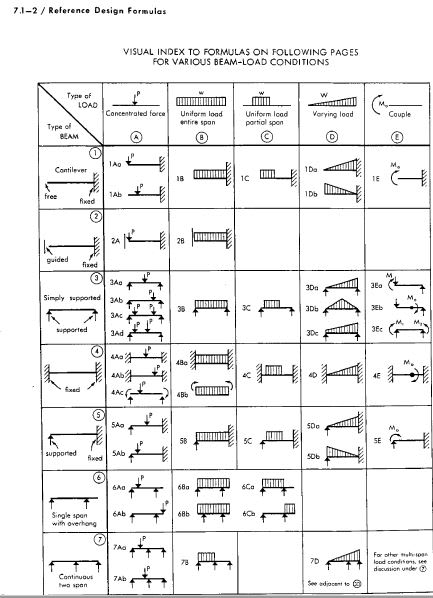### RE: Deflection Equations

Structural engineers should get a feel for the magnitude of deflection to be expected. Study the shape of the bending moment curve and estimate the deflection, even if it turns out to be a waste of time to perform a detailed calculation.

### RE: Deflection Equations

#### Quote (BAretired)

Structural engineers should get a feel for the magnitude of deflection to be expected. Study the shape of the bending moment curve and estimate the deflection, even if it turns out to be a waste of time to perform a detailed calculation.
Estimating the shape of bending moment and deflection curves is not the same as estimating their magnitude. Without calculations (and they will be complicated and long if done by hand for non-trivial cases seldom found in table books such as variable-span or variable-stiffness continuous beams with non-uniform load), the procedure of checking magnitudes is not possible.

EDIT: Of course one can apply the unit load theorem and compute deflection or rotation at discrete points by using the bending diagram, but that also requires a lot of algebra (or alternatively Excel) for any non-trivial structure.

### RE: Deflection Equations

Just to introduce a side-line to the subject, the tools at hand to the engineer can start with the traditional book reference for the classic hand calc, such as Roark. As the problem gets more difficult, other tools start to come in handy, such as Excel. One tool that I have found to be very useful for the case where the problem is more complex, involving aspects such as end restraints, point forces / supports, step moments and / or different shapes of distributed loading and changing cross section, Mathcad has proven to be very useful. Bending moment, section inertia and integral functions can be set up with unknown variables included. The necessary number of equations to solve for the unknowns can be constructed. This obviously is based on first principals(prismatic and non-prismatic). The top of the list would probably be FEA.

### RE: Deflection Equations

#### Quote (EngDM)

Anyone know of a resource to find a ton of different deflection equations? Everything I can find for a UDL not across the whole beam (and not located starting at a support) only gives the moment equation and no deflection.

Or if you know of a good resource where they go over the derivation of the deflection equations. For weird cases I typically use clearcalcs or RISA 2D for quick results, but it would be nice to have a spreadsheet that uses the superimpose method.

Here is a case of a partial uniform load on a beam. It would be good for a spread sheet I think, and you could use a separate line for each different kind of load. Two or three lines should be enough for most beams, but there is no limit. In the end, the spread sheet would sum the values at each station, showing a composite deflection diagram.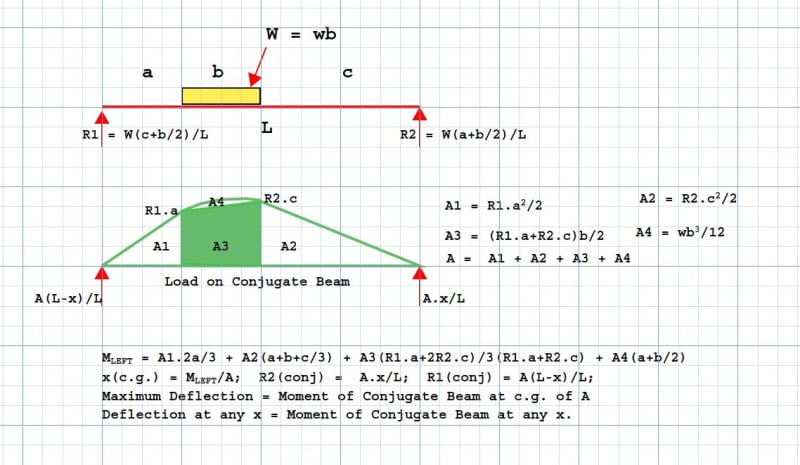### RE: Deflection Equations

I substituted some values and my results are shown below. Have not checked to see if they are reasonable. Will do so in due course.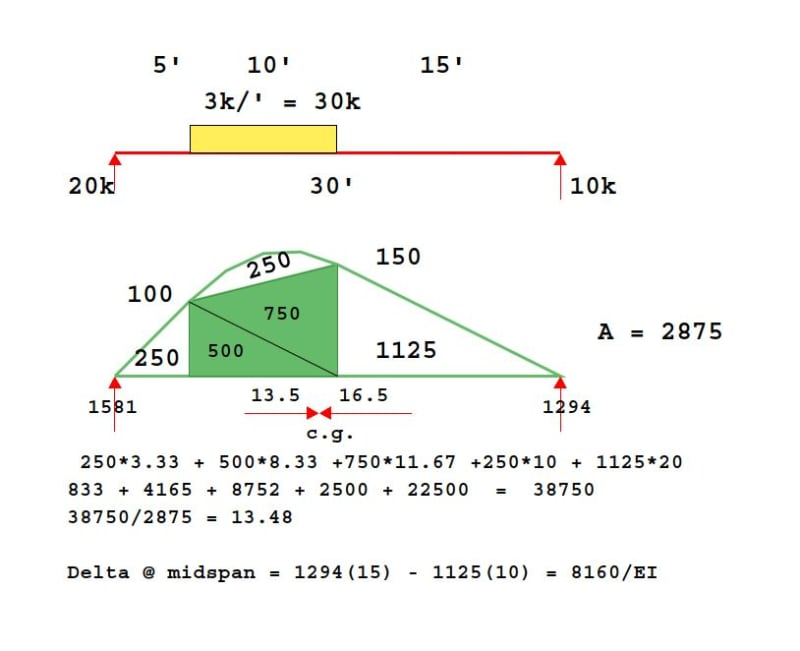### RE: Deflection Equations

For a 30' span with a point load of 30k centred 10' from one end, the moment would be Pab/L = 30*10*20/30 = 200'k. Deflection at point of load would be Pa2b2/3EIl, or 30*(10*20)2/3EI*30= 13,333/EI, considerably more than my result 8160/EI but looks believable.

The method needs more testing, but the OP should be optimistic about using a spreadsheet.

### RE: Deflection Equations

1) I believe that I solved this problem back in 1999 in the course of developing some software. The key to my method is to recognize that any possible uniformly varying load can be treated as the superposition of two triangular loads. For the triangular cases, I used shape functions and integration to get the job done (with MathCAD's help).

2) I would not blame you for doubting the accuracy of these equations. That said, I vetted the crap out of them and actually found bug in S-Frame in the process of doing so. I've also been using these equations for about 20 years in some MathCAD design sheets without incident.

3) Realistically, the hardest part of getting this right is typing out the equations properly.

4) The closed form solutions run wicked fast in MathCAD relative to iterative or discretized approaches which is helpful there.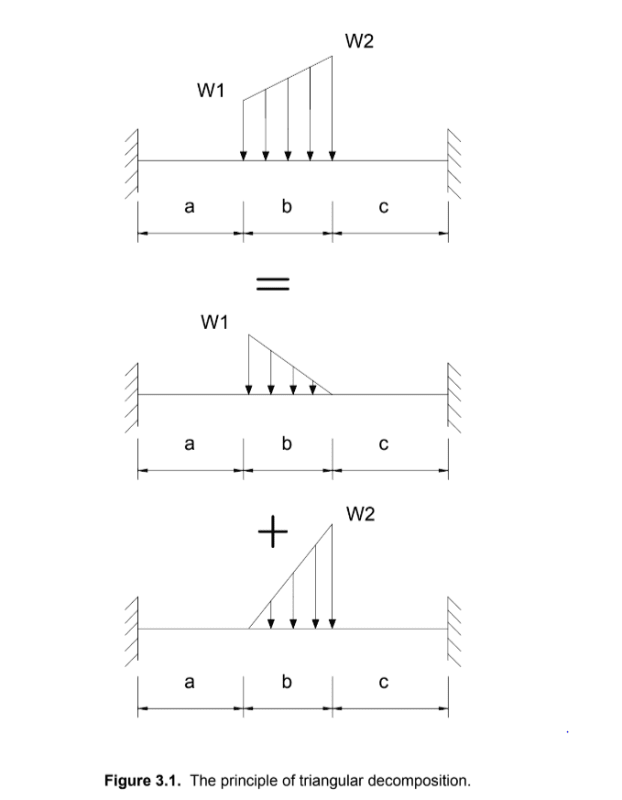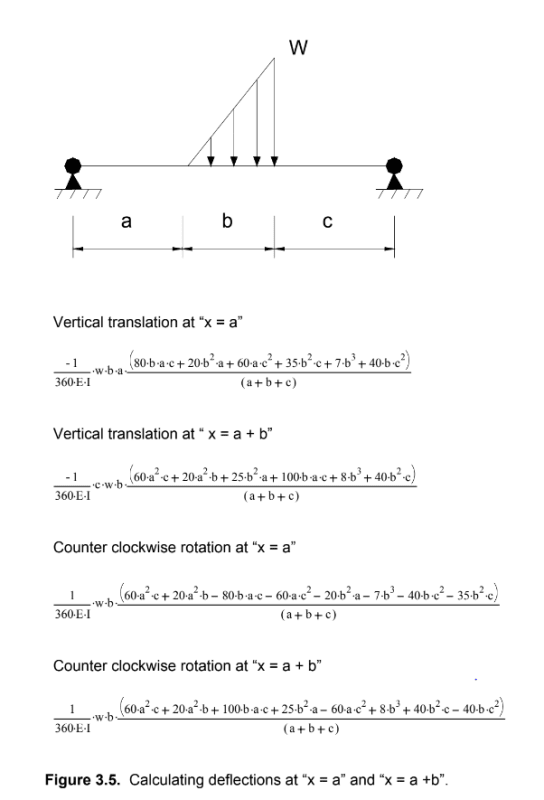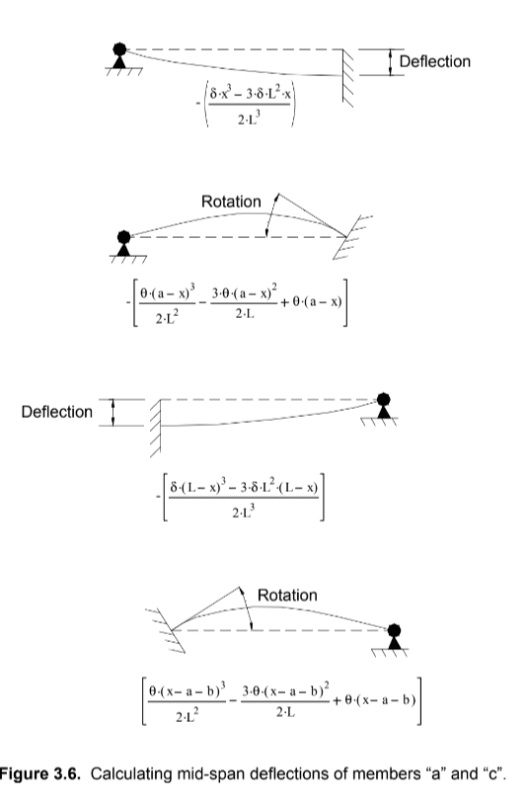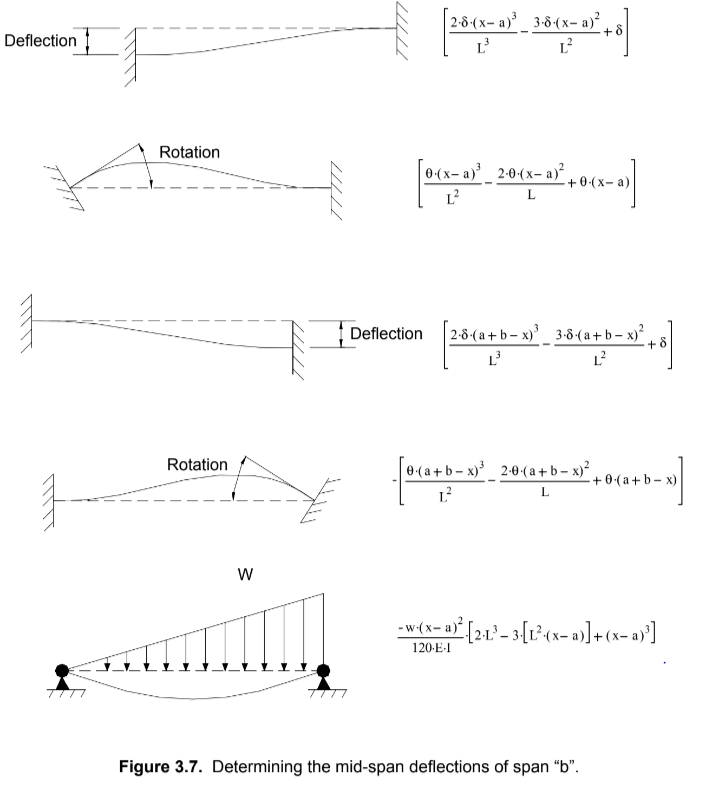### RE: Deflection Equations

Very nice, KootK. There really are a number of ways of solving the problem, but during the course of designing a building, I rarely checked it by calculation because there were usually enough comparisons or obvious clues indicating that the member was code compliant without going through all the work. One method which I used occasionally was Newmarks Numerical Procedures. Even had it included in SlideRuleEra's files...but not any more.

### RE: Deflection Equations

#### Quote (oengineer)

This link contain deflection equations that are not in the steel manual:

Yes thank you, it's quite a good table, but in some cases, deflections are not displayed.### RE: Deflection Equations

yes, many ways to skin cats, and very neat manipulation, but the deflection at the mid-span is not the maximum (though near enough for practical purposes).

I agree with BA, that this is typically not critical for design (so this may be wasted effort).
but I think we should learn things for ourselves (rather than to rely on what we read on the "interwebs").

I would (and have) solved the deflection of the beam mathematically in excel. Solve a couple of simple loadings and you can solve any load and determine the maximum deflection. And with a little more effort different end conditions.

And try things, like compare a full span UDL with a point load, compare a mid-span point load with a point load at "x".

"Hoffen wir mal, dass alles gut geht !"
General Paulus, Nov 1942, outside Stalingrad after the launch of Operation Uranus.

### RE: Deflection Equations

I do like the idea of creating a library of different beam loading conditions, and the obvious first place to look is the readily available hand calc text books and manuals. At some point those places of information will run dry when the problem gets complicated. That’s when you are going to have to choose the right tool for the job, be it Excel, Mathcad or some other (Matlab, Maxima, endless list) and go back to first principals and create the equations yourself. That’s when the fun begins! At some point you may hit the limits of your trusted tool and have to find one more capable. To meet the increasing complexity of your beam analysis cases, you may end up with a library of templates based on various tools.

### RE: Deflection Equations

#### Quote (Stress Eng)

The method applied hasn't been checked or validated.

I ran it in my ConBeamU spreadsheet (using the single span function) and got exactly the same results (to displayed precision).

Using the spreadsheet, if it is important to get the position of maximum deflection and/or bending moment the Excel solver does a good job of finding that. For the deflections I multiplied the deflection by 1E6 in the adjacent column, to save adjusting the solver tolerance, or I could have just set the deflection units to nanometres.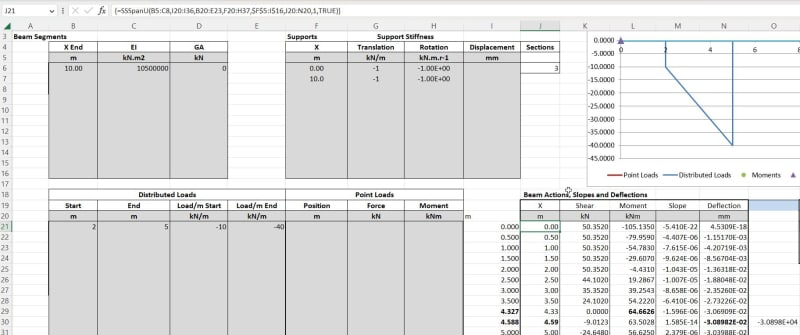I also checked baretired's example and got significantly different results, but I will double-check the units on that one before posting the results.

Doug Jenkins
Interactive Design Services
http://newtonexcelbach.wordpress.com/

### RE: Deflection Equations

Thanks for the feedback IDS, nice to know your results were the same.

### RE: Deflection Equations

Loving the discussion and ways to skin the proverbial cat. If push comes to shove, and if this was in a production environment, I would just run a check in a 2D Frame analysis program versus trying to create a beam sheet that can handle lots (if not all) loading conditions. Or, potentially run SymPy and run with it...YMMV

### RE: Deflection Equations

#### Quote (IDS)

I also checked baretired's example and got significantly different results...

...gulp!

### RE: Deflection Equations

I just checked the Stress-eng example in my FEA software with the beam divided into 10 segments. The magnitude and location of the maximum bending moment agreed exactly, and this is reported in the standard output. The location of the maximum deflection was reported as 4.60 m (compared with 4.588 m) and the magnitude only agreed to 5 significant figures :)

Deflections are found by fitting a cubic shape function to the deflections calculated at beam ends, so it is not surprising that there are small differences compared with our calculations, where we adjusted the beam end locations to find the "exact" maximum deflections.

Note that all these calculations are ignoring shear deflections, which would have a far bigger effect than the 6th significant figure.

BA - I'll post my results later. They were the same order of magnitude as yours :)

Doug Jenkins
Interactive Design Services
http://newtonexcelbach.wordpress.com/

### RE: Deflection Equations

Thanks Doug, I will look forward to see your results. I have just recently heard of the Macauley method of calculating deflections. At first I didn't think it was any different than the double integration method which we learned back in the early 1950's, but it seems there are a couple of new wrinkles which I am not familiar with yet. I'm looking forward to studying it a bit more.

### RE: Deflection Equations

For BAretired's beam:

I set EI to 8160 and got identical results with the spreadsheet and FEA analysis:

With the distributed load: deflection at mid-span = 1.685, maximum moment = 166.7 kip ft.

With the point load at 10 ft: deflection at mid-span = 1.762, maximum moment = 200 kip ft.

Doug Jenkins
Interactive Design Services
http://newtonexcelbach.wordpress.com/

### RE: Deflection Equations

Something is wrong Doug. My last line should read: Delta @ midspan = 1294(15) - 1125(5) = 13,785/EI, not 8160/EI.

I agree with your maximum moments of 167'k and 200'k for the distributed and point load cases respectively, but the deflections can't be right, particularly if they are in feet.

For a maximum moment of 200'k, we could try W18x50 with I of 802in^4. EI=29,000k/in^2*802in^4 = 23.258e6 k-in^2 or 161,514 k-ft^2. Then delta = 13785/161,514 = 0.08535' = 1.02", very nearly L/360, which sounds a little more reasonable.

### RE: Deflection Equations

#### Quote (BAretired)

Something is wrong Doug. My last line should read: Delta @ midspan = 1294(15) - 1125(5) = 13,785/EI, not 8160/EI.

I set the EI to 8160 so the deflection would be 1 foot. Changing to 13,785 I get:
- At mid-span 0.997 ft
= At 13.923 ft (max. deflection point): 1.004 ft.

So that's pretty good :)

Using your realistic EI value I get a maximum deflection of 1.028 in.

By the way the units-aware version of my spreadsheet is very sensitive to the format of non-SI units, and it doesn't give a helpful message if it doesn't like the units, so I'm working on making it friendlier. I will post here when I have finished.

For now, units that work are:
EI: kipf.ft2
Moment: kip ft or kip in

(so EI needs a . between the units, but for moment it has to be a space)

Doug Jenkins
Interactive Design Services
http://newtonexcelbach.wordpress.com/

### RE: Deflection Equations

#### Quote (BAretired)

In most cases, finding deflection is an exercise to meet code requirements after having already selected a beam which has adequate strength. Often, the deflections are not a problem. When that is the case, there is no point in wasting design time performing useless work.

Interesting. 75% of all beams I size are deflection limited. This is residential and light commercial though. So much so, that I check deflection first on most beams.

### RE: Deflection Equations

Do you select a minimum depth of L/20 in the case of a steel beam? If you do, deflection will not be such a common problem. Checking deflection first is definitely ass backwards.

### RE: Deflection Equations

#### Quote (BAretired)

Checking deflection first is definitely ass backwards.

Uh, yeah, NO

Honestly, I can count on the one hand the number of times strength controlled an LVL or steel beam in residential or light commercial so I might as well check deflection first.Bearing stress is the next thing I check for wood construction.

### RE: Deflection Equations

Yeah, I second @XR250. Deflection seems to guide my beam sizes more often than strength.

### RE: Deflection Equations

If that is the case, how do you solve for beam deflections in complicated loading patterns such as described by the OP in this thread, or do you rely entirely on computer programs?

### RE: Deflection Equations

I typically make simplifying assumptions or I use a beam program. Unless I am trying to design to the gnats ass, it is usually good enough. I have done enough beams by hand and then checked them with software that I have a pretty good idea of how i can simplify loadings.

### RE: Deflection Equations

Good answer, XR 250. I did something similar when I was practicing, except there wasn't such a wide choice of software in those days to check hand calculations. I'd like to look further into the Macauley Method just for fun. At first glance, it seems to be a simplification of hand calcs.

### RE: Deflection Equations

I use a beam program and ballpark it with a simplified loading scenario that's covered in the DA6 document or the tables. Meets my needs, I understand the Fermi problems of the results, and still can keep trucking with production work. It would be interesting to know what the OP's original design situation.

### RE: Deflection Equations

The same patch loading solved earlier using Conjugate Beam is solved below using the Macauley Method. It looks like a good way to find deflection when there are many different load types and it should be ideal for a spreadsheet.

The location of maximum deflection could have been solved by setting y' equal to zero, but it would require solving a cubic equation, so deflections were found at three locations. Maximum deflection is seen to be located between 13' and 15' from the left end.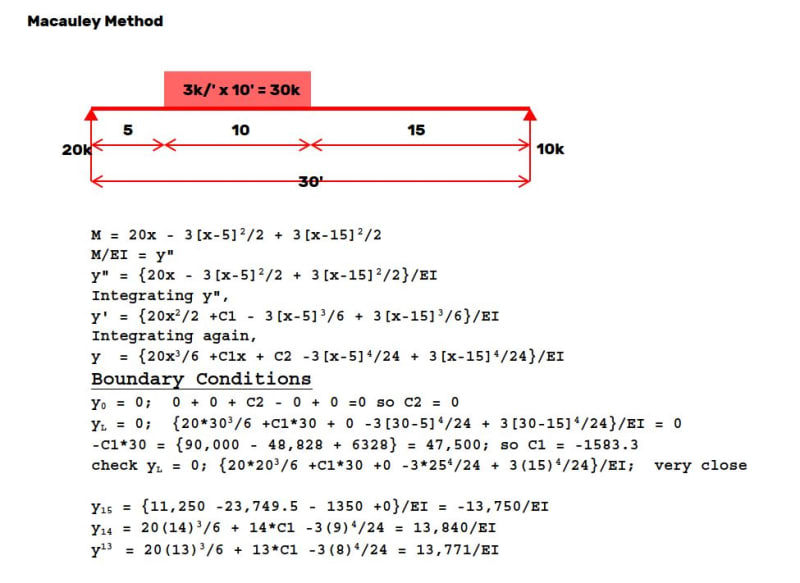### RE: Deflection Equations

Full general derivations via direct integration for Concentrated Moment, Point Load, and linear varying distributed load can be found here: Link

These are derived using a consistent sign convention of positive loading and reactions pointing in the positive y direction, positive moments are counter-clockwise, and positive internal shear points in the negative y direction. This results in beam rotations and deflections consistent with common practice. The derivations assume consistent units across the various inputs. Fixed end moment formulas are also included.

#### Red Flag This Post

Please let us know here why this post is inappropriate. Reasons such as off-topic, duplicates, flames, illegal, vulgar, or students posting their homework.

#### Red Flag Submitted

Thank you for helping keep Eng-Tips Forums free from inappropriate posts.
The Eng-Tips staff will check this out and take appropriate action.

#### Resources

Low-Volume Rapid Injection Molding With 3D Printed Molds
Learn methods and guidelines for using stereolithography (SLA) 3D printed molds in the injection molding process to lower costs and lead time. Discover how this hybrid manufacturing process enables on-demand mold fabrication to quickly produce small batches of thermoplastic parts. Download Now
Examine how the principles of DfAM upend many of the long-standing rules around manufacturability - allowing engineers and designers to place a partâ€™s function at the center of their design considerations. Download Now
Industry Perspective: Education and Metal 3D Printing
Metal 3D printing has rapidly emerged as a key technology in modern design and manufacturing, so itâ€™s critical educational institutions include it in their curricula to avoid leaving students at a disadvantage as they enter the workforce. Download Now

Close Box

# Join Eng-Tips® Today!

Join your peers on the Internet's largest technical engineering professional community.
It's easy to join and it's free.

Here's Why Members Love Eng-Tips Forums:

•Talk To Other Members
• Notification Of Responses To Questions
• Favorite Forums One Click Access
• Keyword Search Of All Posts, And More...

Register now while it's still free!